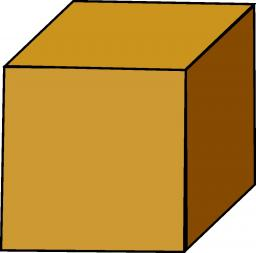# Cube walls

Find the volume and surface area of the cube if the area of one wall is 40cm2.

V =  252.9822 cm3
S =  240 cm2

### Step-by-step explanation:Did you find an error or inaccuracy? Feel free to write us. Thank you!Tips to related online calculators
Tip: Our volume units converter will help you with the conversion of volume units.

#### You need to know the following knowledge to solve this word math problem:

We encourage you to watch this tutorial video on this math problem:

## Related math problems and questions:

• Cube wallsFind the volume and the surface area of the cube if the area of one of its walls is 40 cm2.
• Surface and volume - cubeFind the surface and volume of a cube whose wall diagonal is 5 cm long.
• Cube diagonalsDetermine the volume and surface area of the cube if you know the length of the body diagonal u = 216 cm.
• Cube 1-2-3Calculate the volume and surface area of the cube ABCDEFGH if: a) /AB/ = 4 cm b) perimeter of wall ABCD is 22 cm c) the sum of the lengths of all edges of the cube is 30 cm.
• Cube wallThe surface of the first cube wall is 64 m2. The second cube area is 40% of the surface of the first cube. Determine the length of the edge of the second cube (x).
• Body diagonal - cubeCalculate the surface and cube volume with body diagonal 15 cm long.
• Uboid volumeCalculate the cuboid volume if the walls are 30cm², 35cm², 42cm²
• Cuboid wallsCalculate the cuboid volume if its different walls have an area of 195cm², 135cm², and 117cm².
• Cube wallThe perimeter of one cube wall is 120 meters. Calculate the surface area and the body diagonal of this cube.
• Wall diagonalCalculate the length of wall diagonal of the cube whose surface is 384 cm square.
• Cube diagonalsThe cube has a wall area of 81 cm square. Calculate the length of its edge, wall, and body diagonal.
• Prism XThe prism with the edges of the lengths x cm, 2x cm, and 3x cm has volume 20250 cm3. What is the area of the surface of the prism?
• The cubeThe cube has a surface area of 216 dm2. Calculate: a) the content of one wall, b) edge length, c) cube volume.
• Sphere A2VThe surface of the sphere is 241 mm2. What is its volume?
• Cube surface and volumeThe surface of the cube is 500 cm2, how much cm3 will be its volume?
• Cube wallCalculate the cube's diagonal if you know that one wall's surface is equal to 36 centimeters square. Please also calculate its volume.
• Triangular prismThe triangular prism has a base in the shape of a right triangle, the legs of which is 9 cm and 40 cm long. The height of the prism is 20 cm. What is its volume cm3? And the surface cm2?